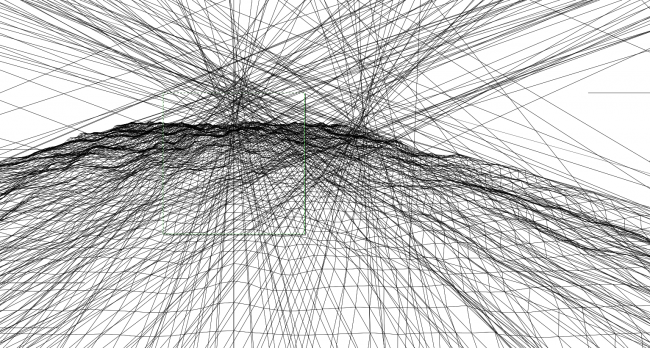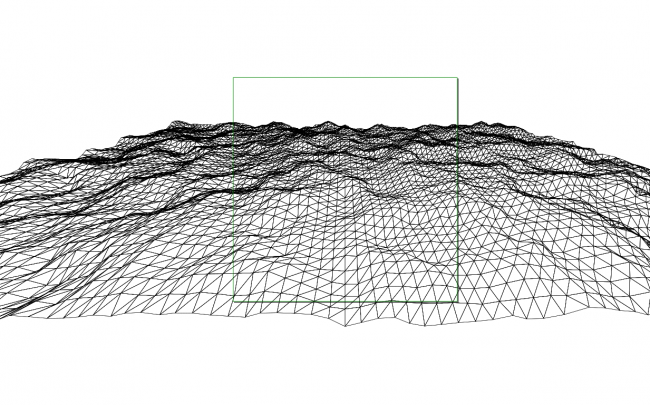#### Howdy, Stranger!

We are about to switch to a new forum software. Until then we have removed the registration on this forum.

# How to save a PDF on key press from a 3D scene in this example?

edited May 2018

I'm trying to add code for saving PDFs on key press, what is the correct way of doing it? Currently saving code doesn't work:

```````
//Stuff for saving stage
import processing.pdf.*;
boolean record = false;

int cols, rows;
int scl = 20;
int w = 2000;
int h = 1600;

float flying = 0;

float[][] terrain;

void setup() {
size(600, 600, P3D);
cols = w / scl;
rows = h/ scl;
terrain = new float[cols][rows];
}

void draw() {
flying -= 0.1;

float yoff = flying;
for (int y = 0; y < rows; y++) {
float xoff = 0;
for (int x = 0; x < cols; x++) {
terrain[x][y] = map(noise(xoff, yoff), 0, 1, 0,100);
xoff += 0.2;
}
yoff += 0.2;
}

background(220);
stroke(255);
noFill();

translate(width/2, height/2+50);
rotateX(PI/3);
translate(-w/2, -h/2);
for (int y = 0; y < rows-1; y++) {
beginShape(TRIANGLE_STRIP);
for (int x = 0; x < cols; x++) {
vertex(x*scl, y*scl, terrain[x][y]);
vertex(x*scl, (y+1)*scl, terrain[x][y+1]);
}
endShape();
}
}

// Trying to save a PDF
void draw() {
PGraphics tmp = null;

if (record) {
tmp = beginRecord(PDF, "render-######.pdf");
}

if (tmp == null) {
H.drawStage();
} else {
PGraphics g = tmp;
boolean uses3D = false;
float alpha = 1;
H.stage().paintAll(g, uses3D, alpha);
}

if (record) {
endRecord();
record = false;
}
}

void keyPressed() {
if (key == 's') {
record = true;
draw();
}
}
`
``````
Tagged:

• edit post, highlight code, press ctrl-o to format code

• two draw()s isn't going to work

what's all the H stuff for? where did you get that code?

• the H is Hype. you don't need it.

the interesting bit are the two `if (record)` blocks. they need moving around the drawing code in your main draw() method

(but that won't work in this case, but make the above changes first and post the new code)

• as described here

https://processing.org/reference/libraries/pdf/index.html

specifically the section titled:

"Single Frame from an Animation (With Screen Display)"

• Shuffled code around a bit thanks to your comments. Now a PDF saves but it's blank, console says: "rotateX() can only be used with a renderer that supports 3D, such as P3D. vertex() with x, y, and z coordinates can only be used with a renderer that supports 3D, such as P3D. Use a version without a z-coordinate instead."

```````
//Stuff for saving stage
import processing.pdf.*;
boolean record = false;

int cols, rows;
int scl = 20;
int w = 2000;
int h = 1600;

float flying = 0;

float[][] terrain;

void setup() {
size(600, 600, P3D);
cols = w / scl;
rows = h / scl;
terrain = new float[cols][rows];
}

void draw() {
PGraphics tmp = null;
flying -= 0.1;

if (record) {
tmp = beginRecord(PDF, "render-######.pdf");
}

float yoff = flying;
for (int y = 0; y < rows; y++) {
float xoff = 0;
for (int x = 0; x < cols; x++) {
terrain[x][y] = map(noise(xoff, yoff), 0, 1, 0,100);
xoff += 0.2;
}
yoff += 0.2;
}

background(220);
stroke(255);
noFill();

translate(width/2, height/2+50);
rotateX(PI/3);
translate(-w/2, -h/2);
for (int y = 0; y < rows-1; y++) {
beginShape(TRIANGLE_STRIP);
for (int x = 0; x < cols; x++) {
vertex(x*scl, y*scl, terrain[x][y]);
vertex(x*scl, (y+1)*scl, terrain[x][y+1]);
}
endShape();
}

// Piece of saving code

if (record) {
endRecord();
record = false;
}
}

// Use keypress so thousands of files aren't created

void keyPressed() {
if (key == 's') {
record = true;
}
}
`
``````
• Yep, because PDFs are 2d...

However, if you scroll down to the section labeled "PDF Files from 3D Geometry (With Screen Display)" and use the raw version then you won't get those errors...

• I replaced beginRecord with beginRaw and endRecord with endRaw according to the "PDF Files from 3D Geometry" section. Renders blank PDF again, this time even without a background color. Console message:

``````'
"X11Util.Display: Shutdown (JVM shutdown: true, open (no close attempt): 2/2, reusable (open, marked uncloseable): 0, pending (open in creation order): 2)
X11Util: Open X11 Display Connections: 2
X11Util: Open: NamedX11Display[:0, 0x7f8c24006aa0, refCount 1, unCloseable false]
X11Util: Open: NamedX11Display[:0, 0x7f8c24018c60, refCount 1, unCloseable false]
Finished.
[Finished in 27.7s]
'
``````
• post the full code. don't make us guess.

• ``````'
//Stuff for saving stage
import processing.pdf.*;
boolean record;

int cols, rows;
int scl = 20;
int w = 2000;
int h = 1600;

float flying = 0;

float[][] terrain;

void setup() {
size(600, 600, P3D);
cols = w / scl;
rows = h / scl;
terrain = new float[cols][rows];
}

void draw() {
// Begin making PDF
if (record) {
beginRaw(PDF, "render-######.pdf");
}

// Do all your drawing here
flying -= 0.1;

float yoff = flying;
for (int y = 0; y < rows; y++) {
float xoff = 0;
for (int x = 0; x < cols; x++) {
terrain[x][y] = map(noise(xoff, yoff), 0, 1, 0,100);
xoff += 0.2;
}
yoff += 0.2;
}

background(22);
stroke(255);
noFill();

translate(width/2, height/2+50);
rotateX(PI/3);
translate(-w/2, -h/2);
for (int y = 0; y < rows-1; y++) {
beginShape(TRIANGLE_STRIP);
for (int x = 0; x < cols; x++) {
vertex(x*scl, y*scl, terrain[x][y]);
vertex(x*scl, (y+1)*scl, terrain[x][y+1]);
}
endShape();
}

// End making PDF
if (record) {
endRaw();
record = false;
}
}

// Use keypress so thousands of files aren't created
// Make PDF once "s" is pressed
void keyPressed() {
if (key == 's') {
record = true;
}
}
'
``````
• If you inflate the PDF and look at the source then the code for the image is there, but there's a lot of extraneous stuff including large triangles drawn between the camera and the landscape. (I think this is the old 3d projection bug). Inkscape in outline mode shows them up.

I think you need to use something other than triangle strips to draw the image if you're saving a pdf, something slower but without the problem. I'll have a look (and post an inkscape pic so you can see what I'm talking about) but it's currently 3am here so...

•here are all the triangles in the pdf. the square is the visible part, the bit in the processing window, the rest is noise. i believe it's cause by this:

``````public class PGraphicsPDF extends PGraphicsJava2D
``````
• This is better. only two lines have changed - it now uses an explicit fill and doesn't draw as many triangles (it's drawing behind the camera which is the problem, if my theory is correct)

``````void draw() {
// Begin making PDF
if (record) {
beginRaw(PDF, "render-######.pdf");
}

// Do all your drawing here
flying -= 0.1;

float yoff = flying;
for (int y = 0; y < rows; y++) {
float xoff = 0;
for (int x = 0; x < cols; x++) {
terrain[x][y] = map(noise(xoff, yoff), 0, 1, 0, 100);
xoff += 0.2;
}
yoff += 0.2;
}

background(22);
stroke(255);
fill(0); // changed from noFill

translate(width / 2, height / 2 + 50);
rotateX(PI / 3);
translate(-w / 2, -h / 2);
for (int y = 0; y < rows - 25; y++) { // changed from rows - 1
beginShape(TRIANGLE_STRIP);
for (int x = 0; x < cols; x++) {
vertex(x * scl, y * scl, terrain[x][y]);
vertex(x * scl, (y + 1) * scl, terrain[x][y + 1]);
}
endShape();
}

// End making PDF
if (record) {
endRaw();
record = false;
}
}
``````

which looks like this in inkscape, much cleaner• Thank you for guiding me through this, so helpful.

How did you extend the scene and found 'public class PGraphicsPDF extends PGraphicsJava2D'? I tried to open the PDF in Sublime Text and in the Chrome browser but didn’t find this line of code. I see the extra triangles on a saved .svg though.

And how did you figure out that those 2 lines (nofill and rows) should be changed to fix it?

• no, the problem is that the PDF writing code is based on the old PGraphicsJava2D and that is, i think, the thing that does a terrible job of clipping lines.

this `PGraphicsPDF extends PGraphicsJava2D` comes from here, deep in the processing source code:

https://github.com/processing/processing/blob/b8936cbd592edcd1620648f1bf9f689176e191f1/java/libraries/pdf/src/processing/pdf/PGraphicsPDF.java#L50

And how did you figure out that those 2 lines (nofill and rows) should be changed to fix it?

the noFill was a guess - nothing was showing up and that's often because you're drawing black on black or drawing invisible triangles so i just fiddled with it

it was obvious (to me, because i've had this problem for years) that there was too much drawing, that things were being drawn behind the camera. changing the rows was the obvious thing to change. the actual -25 value was trial and error, -10 wasn't enough, -50 was too many.

• A slightly shorter link for `public class PGraphicsPDF extends PGraphicsJava2D {`: :\">

https://GitHub.com/processing/processing/blob/master/java/libraries/pdf/src/processing/pdf/PGraphicsPDF.java#L50

• Thank you guys! On the weekend I’ll research the algorithm (Perlin noise?) behind this terrain grid and play with it in Inkscape or Illustrator.

Also found an exciting JS version of the Diamond-Square terrain grid to explore: https://github.com/qiao/fractal-terrain-generator/blob/master/README.md

Can it be launched in Processing like a usual .pde?

• Just checking, but do you know that is js and not java? You can includ it in any html code and it can be run in any browser. You could even use it in the PDE under p5.js mode. Now, taking a wide guess, you could use nashorn

https://forum.processing.org/two/search?Search=nashorn

to run it in Processing java. I haven't done it myself but probly @GoToLoop could tell you if it is possible as he has written most of those post of js in java.

Kf